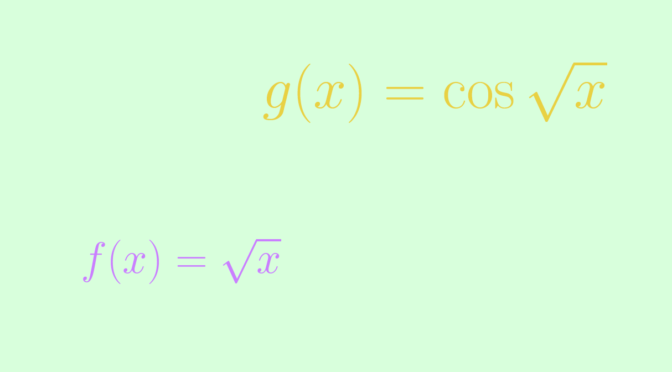# On limit at infinity of functions and their derivatives

We consider continuously differentiable real functions defined on $$(0,\infty)$$ and the limits $\lim\limits_{x \to \infty} f(x) \text{ and } \lim\limits_{x \to \infty} f^\prime(x).$

### A map $$f$$ such that $$\lim\limits_{x \to \infty} f(x) = \infty$$ and $$\lim\limits_{x \to \infty} f^\prime(x) = 0$$

Consider the map $$f : x \mapsto \sqrt{x}$$. It is clear that $$\lim\limits_{x \to \infty} f(x) = \infty$$. As $$f^\prime(x) = \frac{1}{2 \sqrt{x}}$$, we have as announced $$\lim\limits_{x \to \infty} f^\prime(x) = 0$$

### A bounded map $$g$$ having no limit at infinity such that $$\lim\limits_{x \to \infty} g^\prime(x) = 0$$

One idea is to take an oscillating map whose wavelength is increasing to $$\infty$$. Let’s take the map $$g : x \mapsto \cos \sqrt{x}$$. $$g$$ doesn’t have a limit at $$\infty$$ as for $$n \in \mathbb N$$, we have $$g(n^2 \pi^2) = \cos n \pi = (-1)^n$$. However, the derivative of $$g$$ is $g^\prime(x) = – \frac{\sin \sqrt{x}}{2 \sqrt{x}},$ and as $$\vert g^\prime(x) \vert \le \frac{1}{2 \sqrt{x}}$$ for all $$x \in (0,\infty)$$, we have $$\lim\limits_{x \to \infty} g^\prime(x) = 0$$.